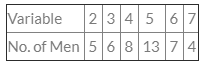# Test: Measures Of Central Tendency And Dispersion- 4

## 40 Questions MCQ Test Quantitative Aptitude for CA CPT | Test: Measures Of Central Tendency And Dispersion- 4

Description
Attempt Test: Measures Of Central Tendency And Dispersion- 4 | 40 questions in 40 minutes | Mock test for CA Foundation preparation | Free important questions MCQ to study Quantitative Aptitude for CA CPT for CA Foundation Exam | Download free PDF with solutions
QUESTION: 1

Solution:
QUESTION: 2

Solution:
QUESTION: 3

### For the values of a variable 5, 2, 8, 3, 7, 4 the median is

Solution:
QUESTION: 4

If the class interval is open-end then it is difficult to find

Solution:
QUESTION: 5

The class having maximum frequency is called

Solution:
QUESTION: 6Mode is

Solution:
QUESTION: 7

The sum of the squares of the deviations of the variable is __________ when taken about A.M

Solution:
QUESTION: 8

The abscissa of the maximum frequency in the frequency curve is the

Solution:
QUESTION: 9

The A.M of 1, 3, 5, 6, x, 10 is 6. The value of x is

Solution:

(1+3+5+6+x+10)/6 = 6

(25+x)/6 = 6

x = 36-25

x = 11

QUESTION: 10

If the A.M of any distribution be 25 & one term is 18. Then the deviation of 18 from A.M is

Solution:
QUESTION: 11

For finding A.M in Step – deviation method, the class intervals should be of

Solution:
QUESTION: 12

(n+1)/2 th term is median if n is

Solution:
QUESTION: 13

The value of a variate that occur most often is called

Solution:
QUESTION: 14

The G.M of 2 & 8 is

Solution:
QUESTION: 15

First Quartile lies in the class interval of the

Solution:
QUESTION: 16

Which one is true –

Solution:
QUESTION: 17

Semi-quartile range is one-fourth of the range in a normal symmetrical distribution.

Solution:
QUESTION: 18

If each item is reduced by 15 A.M is

Solution:
QUESTION: 19

If the variables are increased or decreased by the same proportion, the standard deviation changes by

Solution:
QUESTION: 20

If the variables are increased or decreased by the same amount the standard deviation is

Solution:
QUESTION: 21

Coefficient of Quartile Deviation is equal to

Solution:
QUESTION: 22

If each item is reduced by 20, the standard deviation

Solution:
QUESTION: 23

The mean of the 1st n natural no. is

Solution:
QUESTION: 24

Coefficient of variation is a relative measure of

Solution:
QUESTION: 25

Coefficient of variation is equal to

Solution:
QUESTION: 26

Quartile deviation is based on the

Solution:
QUESTION: 27

Relative measures of dispersion make deviations in similar units comparable.

Solution:
QUESTION: 28

S.D is less than Mean deviation

Solution:
QUESTION: 29

If each item is reduced by 10, the range is

Solution:
QUESTION: 30

Coefficient of variation is independent of  the unit of measurement.

Solution:
QUESTION: 31

Whole frequency table is needed for the calculation of

Solution:
QUESTION: 32

Mean is an absolute measure & Standard deviation is based upon it. Therefore standard deviation is a relative measure.

Solution:
QUESTION: 33

For a moderately non-symmetrical distribution, Quartile deviation = Standard deviation /3

Solution:
QUESTION: 34

If all the values are multiplies by the same quantity, the __________ & _________ also would be multiple of the same quantity.

Solution:
QUESTION: 35

For a moderately non-symmetrical distribution, Probable error of standard deviation =Standard deviation/3

Solution:
QUESTION: 36

When all the values are equal then variance & standard deviation would be

Solution:
QUESTION: 37

Coefficient of standard deviation is

Solution:
QUESTION: 38

If the same amount is added to or subtracted from all the values, the mean shall increase or decrease by the __________ amount

Solution:
QUESTION: 39

In _________ range has the greatest use.

Solution:
QUESTION: 40

For a moderately non-symmetrical distribution, Mean deviation = 4/5 of standard deviation

Solution:Use Code STAYHOME200 and get INR 200 additional OFF Use Coupon Code